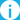Sie haben Javascript deaktiviert!
Sie haben versucht eine Funktion zu nutzen, die nur mit Javascript möglich ist. Um sämtliche Funktionalitäten unserer Internetseite zu nutzen, aktivieren Sie bitte Javascript in Ihrem Browser.Diese Seite ist nicht in Deutsch verfügbarData Center Building O

| Paderborn Center for Parallel Computing

# Efficient Computation of Inverse Matrix Roots

Our article A General Algorithm to Calculate the Inverse Principal p-th Root of Symmetric Positive Definite Matrices has been accepted for publication in the Communications in Computational Physics (CICP). This article is a joint work by the research groups of three PC² board members from the Departments of Chemistry, Computer Science and Mathematics. In the article, we describe an iteration scheme for the calculation of inverse roots of matrices and prove and analyze its convergence under specific conditions. The proposed method allows trading of the number of iterations required to obtain a result against the computational effort within each iteration. The approach is a generalization of previously published methods such as the hyperpower method by Altman (1960) and the iteration scheme proposed by Bini, Higham and Meini (2005).

Inverse matrices and inverse roots are required in different applications, such as preconditioning and solving generalized eigenvalue problems, in particular to solve Schrodinger and Maxwell equations. In earlier work [1,2,3] we have shown the great potential of Approximate Computing techniques for these application areas, as results are not always required to be exact. These techniques aim at increasing the energy-efficiency of algorithms, opposing the ever-growing energy demands of HPC systems. Iterative methods, as the one described in our article, are especially well suited for the use of approximative methods, as introduced errors can be corrected in subsequent iterations.

An early, non-final preprint of our article is available from https://arxiv.org/abs/1703.02456

Abstract

We address the general mathematical problem of computing the inverse p-th root of a given matrix in an efficient way. A new method to construct iteration functions that allow calculating arbitrary p-th roots and their inverses of symmetric positive definite matrices is presented. We show that the order of convergence is at least quadratic and that adjusting a parameter q leads to an even faster convergence. In this way, a better performance than with previously known iteration schemes is achieved. The efficiency of the iterative functions is demonstrated for various matrices with different densities, condition numbers and spectral radii.

 Lass, Kühne, Plessl: Using Approximate Computing in Scientific Codes, Workshop on Approximate Computing. Oct. 2016.

 Lass, Kühne, Plessl: Using Approximate Computing for the Calculation of Inverse Matrix p-th Roots, IEEE Embedded Systems Letters. Accepted for publication.

 Lass, Kühne, Plessl: A Massively Parallel Algorithm for the Approximate Calculation of Inverse p-th Roots of Large Sparse Matrices, Preprint.

Die Universität der Informationsgesellschaft